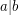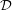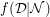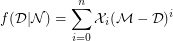# Distribution and upper bound of mimic numbers

 Importance: Medium ✭✭
 Author(s): Bhattacharyya, M.
 Subject: Number Theory » Analytic Number Theory
 Keywords: Divisibility mimic function mimic number
 Recomm. for undergrads: yes
 Prize: Not yet declared
 Posted by: facility_cttb@i... on: June 20th, 2009
Problem

Let the notationdenote ''divides''. The mimic function in number theory is defined as follows .

Definition   For any positive integerdivisible by, the mimic function,, is given by,By using this definition of mimic function, the mimic number of any non-prime integer is defined as follows .

Definition   The numberis defined to be the mimic number of any positive integer, with respect to, for the minimum value of which.

Given these two definitions and a positive integer, find the distribution of mimic numbers of those numbers divisible by.

Again, find whether there is an upper bound of mimic numbers for a set of numbers divisible by any fixed positive integer.

## Bibliography

* Malay Bhattacharyya, Sanghamitra Bandyopadhyay and U Maulik, Non-primes are recursively divisible, Acta Universitatis Apulensis 19 (2009).

* indicates original appearance(s) of problem.